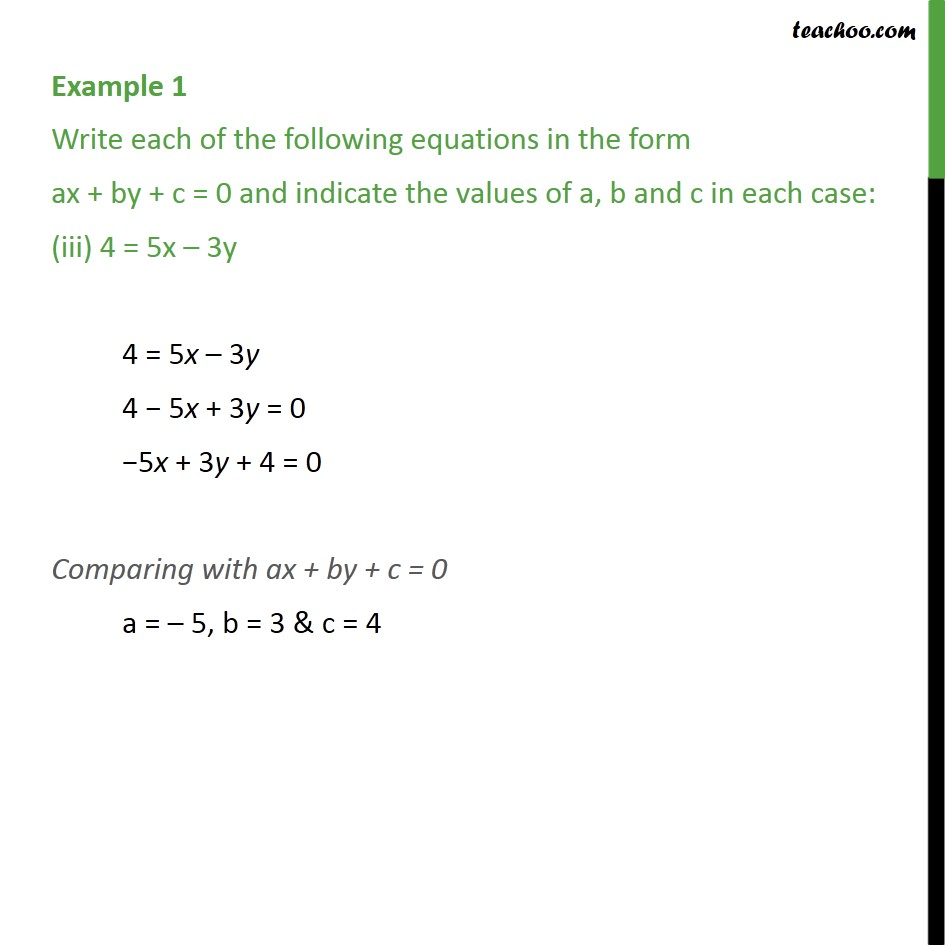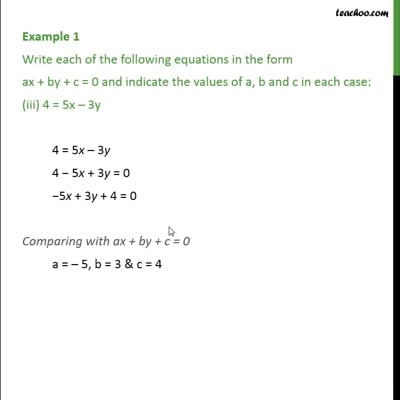Examples

Chapter 4 Class 9 Linear Equations in Two Variables
Serial order wiseThis video is only available for Teachoo black users

### Transcript

Example 1 Write each of the following equations in the form ax + by + c = 0 and indicate the values of a, b and c in each case: (iii) 4 = 5x – 3y 4 = 5x – 3y 4 − 5x + 3y = 0 −5x + 3y + 4 = 0 Comparing with ax + by + c = 0 a = – 5, b = 3 & c = 4